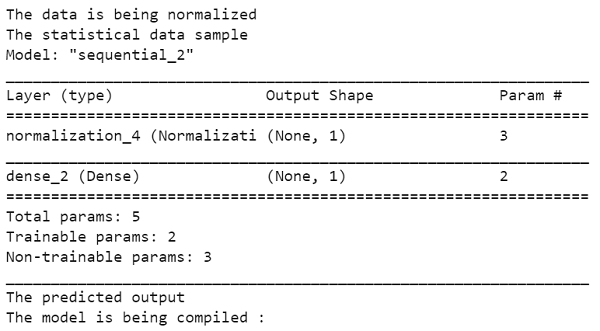# How can predictions be made about the fuel efficiency with Auto MPG dataset using TensorFlow?

PythonServer Side ProgrammingProgramming

Tensorflow is a machine learning framework that is provided by Google. It is an open−source framework used in conjunction with Python to implement algorithms, deep learning applications and much more. It is used in research and for production purposes. It has optimization techniques that help in performing complicated mathematical operations quickly.

This is because it uses NumPy and multi−dimensional arrays. These multi-dimensional arrays are also known as ‘tensors’. The framework supports working with deep neural network. It is highly scalable, and comes with many popular datasets. It uses GPU computation and automates the management of resources. It comes with multitude of machine learning libraries, and is well-supported and documented. The framework has the ability to run deep neural network models, train them, and create applications that predict relevant characteristics of the respective datasets.

The ‘tensorflow’ package can be installed on Windows using the below line of code −

pip install tensorflow

Tensor is a data structure used in TensorFlow. It helps connect edges in a flow diagram. This flow diagram is known as the ‘Data flow graph’. Tensors are nothing but multidimensional array or a list. They can be identified using three main attributes −

The aim behind a regression problem is to predict the output of a continuous or discrete variable, such as a price, probability, whether it would rain or not and so on.

The dataset we use is called the ‘Auto MPG’ dataset. It contains fuel efficiency of 1970s and 1980s automobiles. It includes attributes like weight, horsepower, displacement, and so on. With this, we need to predict the fuel efficiency of specific vehicles.

We are using the Google Colaboratory to run the below code. Google Colab or Colaboratory helps run Python code over the browser and requires zero configuration and free access to GPUs (Graphical Processing Units). Colaboratory has been built on top of Jupyter Notebook.

Following is the code snippet −

## Example

hrspwr = np.array(train_features['Horsepower'])
print("The data is being normalized")
hrspwr_normalizer = preprocessing.Normalization(input_shape=[1,])

hrspwr_model = tf.keras.Sequential([
hrspwr_normalizer,
layers.Dense(units=1)
])
print("The statistical data sample ")
hrspwr_model.summary()
print("The predicted output ")
hrspwr_model.predict(hrspwr[:7])
print("The model is being compiled : ")
hrspwr_model.compile(
loss='mean_absolute_error')

## Output## Explanation

• The ‘MPG’ value from ‘Horsepower’ needs to be predicted.

• A Keras model is trained by defining the architecture of the model.

• The model defined here is a ‘sequential’ model. It indicates a sequence of steps.

• First, the ‘horsepower’ input is normalized.

• The linear transformation (y= mx + b) is applied which will produce an output with the help of dense layer ‘layers.Dense’.

• The ‘horsepower’ normalization layer is created.